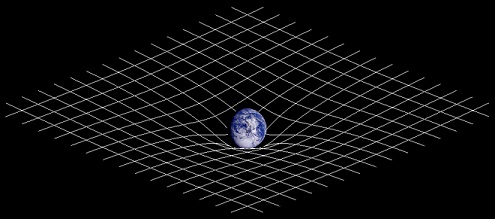# Difference Between Gravity and Gravitation

## Main Difference – Gravity vs. Gravitation

The terms gravity and gravitation both describe the phenomenon which causes masses to attract each other. Both terms are widely used interchangeably, and it is more or less permissible to do so. However, in some fields of study, the difference between gravity and gravitation is more prominent. In these situations, the main difference between gravity and gravitation is that gravitation describes the attractive force between any two masses while gravity specifically describes the resultant force with which an object is attracted towards the Earth.

## What is Gravitation

Gravitation refers to one of the four fundamental forces in physics, responsible for the attraction between masses.  The other three fundamental forces are the strong nuclear force, the weak nuclear force, and electromagnetism. In comparison to these other three forces, gravity is certainly the “odd one out”. While there is a single theory called the grand unified theory which describes the other three forces, gravity alone is explained by Einstein’s general relativity. In addition, the force of gravity is much weaker compared to the other three fundamental forces.

According to the general theory of relativity, masses cause the fabric of space-time around them to be distorted. Any other mass coming closer will experience the warping of space-time, and this is what appears to us as an attractive force between two masses.In general relativity, gravity is thought to come from the warped space-time around massive objects.

However, the general theory of relativity is quite complex. Newton’s law of gravitation is a less complex, but “good enough” approximation to calculate the force of gravitational attraction between different masses. This law states that the gravitational force between two bodies is directly proportional to the product of their masses, and inversely proportional to the square of the separation between them. I.e. if two bodies having masses$m_1$ and$m_2$ are separated by a distance$r$, then the gravitational attraction between them is given by:$F=\frac{Gm_1m_2}{r^2}$

where$G$ is a physical constant known as the gravitational constant, given by$G=6.67\times 10^{-11} \mathrm{\:m^3\:kg^{-1}\:s^{-2}}$.

## What is Gravity

Gravity refers to the resultant force with which an object is attracted towards Earth, for example, the force that the Earth exerts on a ball that makes it fall down. Indeed, gravitation is the main cause for this attraction. However, the Earth is also rotating, and the effects of Earth’s rotation should also be included when calculating gravity.

## Difference Between Gravity and Gravitation

### What it Means

Gravitation refers to the attractive force between two masses in general.

Gravity refers to the resultant force between a mass and Earth. The resultant force also accounts for other forces present in the system, for instance, the force on the mass due to the Earth’s rotation.

Image Courtesy

“Illustration of spacetime curvature.” by User:Johnstone (Created using an image of planet earth from NASA’s Galileo spacecraft.) [CC BY-SA 3.0], via Wikimedia Commons